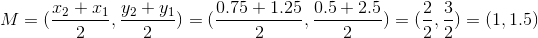Example Questions

Example Question #3 : Midpoint Formula

Find the coordinates for the midpoint of the line segment that spans from (1, 1) to (11, 11).

(7, 7)

(5, 6)

(5, 5)

(6, 5)

(6, 6)

(6, 6)

Explanation:

The correct answer is (6, 6). The midpoint formula is ((x+ x2)/2),((y+ y2)/2) So 1 + 11 = 12, and 12/2 = 6 for both the x and y coordinates.

Example Question #4 : Midpoint Formula

What is the midpoint between the points (–1, 2) and (3, –6)?

(3,1)

(1,2)

(–1,2)

(–2,–1)

(1,–2)

(1,–2)

Explanation:

midpoint = ((x1 + x2)/2, (y1 + y2)/2)

= ((–1 + 3)/2, (2 – 6)/2)

= (2/2, –4/2)

= (1,–2)

Example Question #5 : Midpoint Formula

A line segment connects the points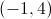and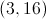. What is the midpoint of this segment?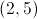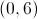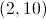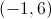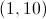Explanation:

To solve this problem you will need to use the midpoint formula: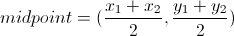Plug in the given values for the endpoints of the segment:and.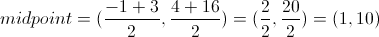Example Question #6 : Midpoint Formula

What is the midpoint between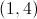and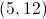?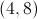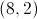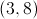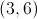Explanation:

The midpoint is the point halfway between the two endpoints, so sum up the coordinates and divide by 2: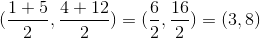Example Question #1 : How To Find The Midpoint Of A Line Segment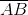has endpoints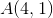and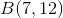What is the midpoint of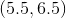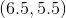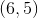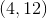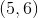Explanation:

The midpoint is simply the point halfway between the x-coordinates and halfway between the y-coordinates:

Sum the x-coordinates and divide by 2: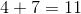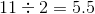Sum the y-coordinates and divide by 2: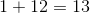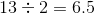Therefore the midpoint is (5.5, 6.5).

Example Question #2 : How To Find The Midpoint Of A Line Segment

What is the mid point of a linear line segment that spans from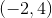to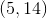?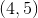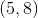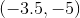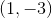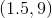Explanation:

To find the midpoint of a line segment, use this formula: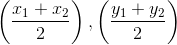Therefore, the midpoint of the given line segment is: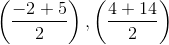Example Question #3 : How To Find The Midpoint Of A Line Segment

Find the midpoint of the line segment with endpoints (1,3) and (5,7).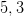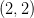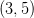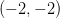Explanation:

To solve, simply use the midpoint formula as outlined below.

Given,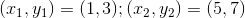Thus,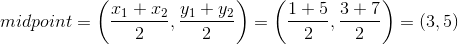Example Question #4 : How To Find The Midpoint Of A Line Segment

Find the midpoint of a line segment with end points (1,3) and (11,3).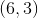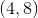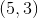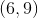Explanation:

To solve, simply realize you are on a horizantal line, so you just need to find the distance betweent he two x coordants and find half way between them. Thus,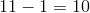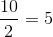Thus, the midpoint is at (1+5,3) which is (6,3).

Example Question #11 : Midpoint Formula

What is the midpoint of a line segment that begins at (0, -1) and ends at (4, 10)?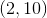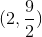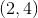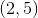Explanation:

The midpoint of a line segment can be found by averaging the x-values and y-values of the given ordered pairs. In other words,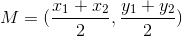.

Take the average of the given x- and y-values of our ordered pairs.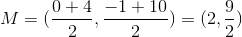Example Question #12 : Midpoint Formula

Find the midpoint between the point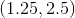and the center of the given circle.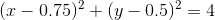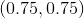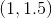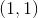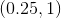Explanation:

Remember that the general equation for a circle with center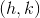and radiusis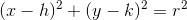With this in mind, the center of our circle is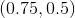To find the midpoint between our two points, use the midpoint formula.